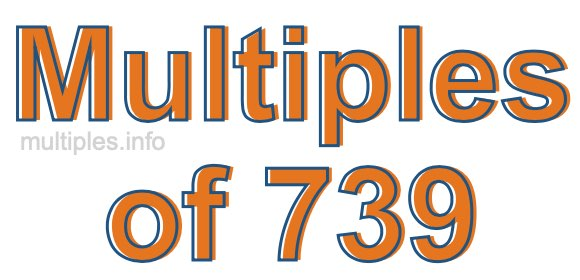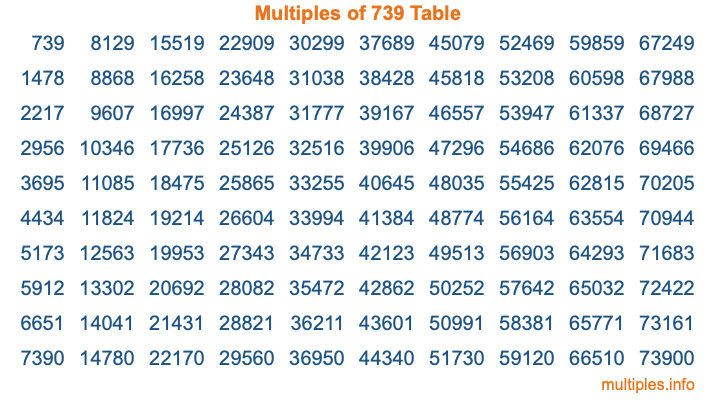Multiples of 739Welcome to the Multiples of 739 page. Here we will first teach you everything you will ever need to know about the multiples of 739, and then give you a study guide summary of everything we taught you to make sure you remember it all. Use this page to look up facts and learn information about the multiples of 739. This page will make you a multiples of seven hundred thirty-nine expert!

Definition of Multiples of 739
Multiples of 739 are all the numbers that when divided by 739 equal an integer. Each of the multiples of 739 are called a multiple. A multiple of 739 is created by multiplying 739 by an integer.

Therefore, to create a list of multiples of 739, you start with 1 multiplied by 739, then 2 multiplied by 739, then 3 multiplied by 739, and so on for as long as you want. Thus, the list of the first five multiples of 739 is 739, 1478, 2217, 2956, and 3695. To see a larger list of multiples of 739, see the printable image of Multiples of 739 further down on this page. We also have a category where you can choose any nth multiple of 739.

Multiples of 739 Checker
The Multiples of 739 Checker below checks to see if any number of your choice is a multiple of 739. In other words, it checks to see if there is any number (integer) that when multiplied by 739 will equal your number. To do that, we divide your number by 739. If the the quotient is an integer, then your number is a multiple of 739.

Is  a multiple of 739?

Least Common Multiple of 739 and ...
A Least Common Multiple (LCM) is the lowest multiple that two or more numbers have in common. This is also called the smallest common multiple or lowest common multiple and is useful to know when you are adding our subtracting fractions. Enter one or more numbers below (739 is already entered) to find the LCM.

Check out our LCM Calculator if you need more details about the Least Common Multiple or if you need the LCM for different numbers for adding and subtraction fractions.

nth Multiple of 739
As we stated above, 739 is the first multiple of 739, 1478 is the second multiple of 739, 2217 is the third multiple of 739, and so on. Enter a number below to find the nth multiple of 739.

th multiple of 739

Multiples of 739 vs Factors of 739
739 is a multiple of 739 and a factor of 739, but that is where the similarities end. All postive multiples of 739 are 739 or greater than 739. All positive factors of 739 are 739 or less than 739.

Below is the beginning list of multiples of 739 and the factors of 739 so you can compare:

Multiples of 739: 739, 1478, 2217, 2956, 3695, etc.

Factors of 739: 1, 739

As you can see, the multiples of 739 are all the numbers that you can divide by 739 to get a whole number. The factors of 739, on the other hand, are all the whole numbers that you can multiply by another whole number to get 739.

It's also interesting to note that if a number (x) is a factor of 739, then 739 will also be a multiple of that number (x).

Multiples of 739 vs Divisors of 739
The divisors of 739 are all the integers that 739 can be divided by evenly. Below is a list of the divisors of 739.

Divisors of 739: 1, 739

The interesting thing to note here is that if you take any multiple of 739 and divide it by a divisor of 739, you will see that the quotient is an integer.

Multiples of 739 Table
Below is an image of the first 100 multiples of 739 in a table. The table is in chronological order, column by column. The first column has the first ten multiples of 739, the second column has the next ten multiples of 739, and so on.The Multiples of 739 Table is also referred to as the 739 Times Table or Times Table of 739. You are welcome to print out our table for your studies.

Negative Multiples of 739
Although not often discussed or needed in math, it is worth mentioning that you can make a list of negative multiples of 739 by multiplying 739 by -1, then by -2, then by -3, and so on, to get the following list of negative multiples of 739:

-739, -1478, -2217, -2956, -3695, etc.

Multiples of 739 Summary
Below is a summary of important Multiples of 739 facts that we have discussed on this page. To retain the knowledge on this page, we recommend that you read through the summary and explain to yourself or a study partner why they hold true.

There are an infinite number of multiples of 739.

A multiple of 739 divided by 739 will equal a whole number.

739 divided by a factor of 739 equals a divisor of 739.

The nth multiple of 739 is n times 739.

The largest factor of 739 is equal to the first positive multiple of 739.

739 is a multiple of every factor of 739.

739 is a multiple of 739.

A multiple of 739 divided by a divisor of 739 equals an integer.

739 divided by a divisor of 739 equals a factor of 739.

Any integer times 739 will equal a multiple of 739.

Multiples of a Number
Here you can get the multiples of another number, all with the same attention to detail as we did for multiples of 739 on this page.

Multiples of
Multiples of 740
Did you find our page about multiples of seven hundred thirty-nine educational? Do you want more knowledge? Check out the multiples of the next number on our list!